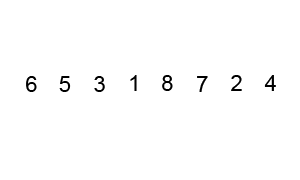Categories

# Basic programming algorithm: Bubble Sort

Bubble sort is one of the most basic sorting algorithms in taught in computer science classes. It will order values in a list from smallest to largest passing several times through the list comparing two items at a time and reordering them. This algorithm is not very efficient with large lists because it has to evaluate every member of the list several times. The longer the list, the more it will take the algorithm to finish. In big-O notation, this algorithm’s efficiency is represented by: O(n2)

An explanation of bubble sort from Wikipedia:

Bubble sort, sometimes incorrectly referred to as sinking sort, is a simple sorting algorithm that works by repeatedly stepping through the list to be sorted, comparing each pair of adjacent items and swapping them if they are in the wrong order. The pass through the list is repeated until no swaps are needed, which indicates that the list is sorted. The algorithm gets its name from the way smaller elements “bubble” to the top of the list. Because it only uses comparisons to operate on elements, it is a comparison sort. Although the algorithm is simple, most of the other sorting algorithms are more efficient for large lists.While passing through the list, compare two elements, if the second one is bigger than the first, swap their positions in the list. Continue going through the list, comparing the next elements in the same way. Go through the list until no more swaps take place.

Pseudocode:

``````while not sorted:
sorted = True
for iterator = 1 to length_of_list - 1 do:
check if pair is out of order:
if list[iterator] is bigger than list[iterator+1] then
swap them:
temporal_value = list[iterator]
list[iterator] = list[iterator+1]
list[iterator+1] = temporal_value
sorted = False
``````

PHP code example:

``````/**
* Sort an array using bubble sort algorithm
* @param array \$list The list to be sorted
* @return array The sorted array
*/
function bubbleSort(\$list) {
while(!\$sorted){
\$sorted = True;
foreach(\$list as \$key => \$value) {
if(\$list[\$key] > \$list[\$key+1]) {
\$temp = \$list[\$key+1];
\$list[\$key+1] = \$list[\$key];
\$list[\$key] = \$temp;
\$sorted = False;
}
}
}
return \$list;
}
``````

Python code example:

``````function bubbleSort(a_list):
""" Sort an array using bubble sort algorithm """
sorted = False
length = len(a_list)
while not sorted:
sorted = True
for position in range(length):
if a_list[position] > a_list[position+1]:
# Swap values
a_list[position], a_list[position+1] = a_list[position+1], a_list[position]
sorted = False
return a_list
``````

There is another way to write the bubble sort, by shrinking the list by one element after every pass. If you see the animated graphic above, after each pass, the last number is already in its correct place, so you don’t have to iterate over those every subsequent time. You can discard the last element each time, making the algorithm faster.

An example of this version in Python code would look like this:

``````function betterBubbleSort(a_list):
""" Sort an array using bubble sort algorithm """
length = len(a_list)
while length:
for position in range(length):
if a_list[position] > a_list[position+1]:
# Swap values
a_list[position], a_list[position+1] = a_list[position+1],a_list[position]
length -= 1
return a_list
``````

Do you have other ways of writing the bubble sort? Share them in the comments section below.## By Gabriel Saldaña

Gabriel Saldaña is a web developer, photographer and free software advocate. Connect with him on and Twitter

This site uses Akismet to reduce spam. Learn how your comment data is processed.